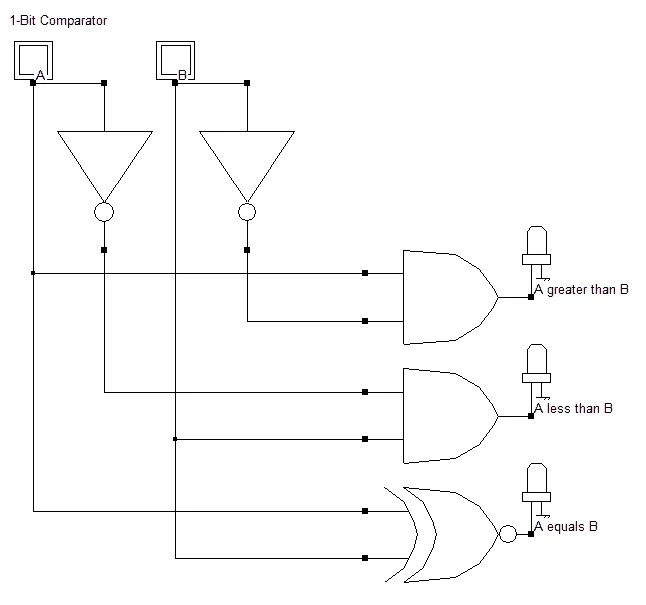View Course Path

# VHDL code for comparator using behavioral method – full code and explanation

In this post, we will take a look at implementing the VHDL code for a comparator using behavioral modeling architecture. First, we will take a look at the logic circuit of the comparator. Then we will understand its behavior using its truth table. And then, we will understand the syntax. In addition to all that, we will also verify our RTL synthesis using a testbench. We will also synthesize the RTL schematic and the simulation waveforms. For the full code, scroll down.

Contents

## Explanation of the VHDL code for comparator using behavioral method. How does the code work?

A comparator is a combinational logic circuit that compares two inputs and gives an output that indicates the relationship between them. There are three outputs.

• An output that indicates if number A is greater than number B.
• An output that indicates if it’s smaller.
• And finally, an output that indicates if the two numbers are equal.

Let’s take a look at its logic circuit for some clarity.

### Logic circuit for 1-bit comparator

As we can see, the 1-bit comparator has two inputs and three outputs.### Truth table for 1-bit comparator

 A B A>B A

Through the medium of writing the VHDL code for this logic circuit, we will understand an application of the case statements. We can use these Case statements because we are using behavioral architecture.

Generally, throughout this course on VHDL programming, we have mentioned that when dealing with truth tables, it is easier to define the entities as vectors. However, in this particular program, we will define the outputs as scalars.

The reason is that either way, the total lines of code that we will be writing will remain unchanged. So let’s take a look at the syntax for the entity-architecture pair and the `begin` statements specific to the behavioral model.

```entity COMPARATOR_SOURCE is
Port ( A : in  STD_LOGIC_VECTOR (1 downto 0);
G,L,E : out  STD_LOGIC);
end COMPARATOR_SOURCE;

architecture Behavioral of COMPARATOR_SOURCE is
```

First off, we will set all the outputs to zero. We will use if-elsif statements to implement the logic.

```begin
process (A)
begin

G <= '0';
L <= '0';
E <= '0';```
```if (A(0) < A(1)) then
L <= '1';

elsif (A(0) > A(1)) then
G <= '1';

else
E <= '1';
end if;```

## VHDL code for comparator using behavioral method

```library IEEE;
use IEEE.STD_LOGIC_1164.ALL;
use IEEE.STD_LOGIC_ARITH.ALL;
use IEEE.STD_LOGIC_UNSIGNED.ALL;

entity COMPARATOR_SOURCE is
Port ( A : in  STD_LOGIC_VECTOR (1 downto 0);
G,L,E : out  STD_LOGIC);
end COMPARATOR_SOURCE;

architecture Behavioral of COMPARATOR_SOURCE is

begin
process (A)
begin

G <= '0';
L <= '0';
E <= '0';```
```if (A(0) < A(1)) then
L <= '1';

elsif (A(0) > A(1)) then
G <= '1';

else
E <= '1';
end if;
end process;
end Behavioral;

```

## Testbench

We will use the testbench type that uses process statements. Here’s a complete guide on writing VHDL testbenches.

```library IEEE;
use IEEE.STD_LOGIC_1164.ALL;
use IEEE.STD_LOGIC_ARITH.ALL;
use IEEE.STD_LOGIC_UNSIGNED.ALL;

entity comparator_tb is
end entity;

architecture tb of comparator_tb is
component COMPARATOR_SOURCE is
Port ( A : in STD_LOGIC_VECTOR (1 downto 0);
G,L,E : out STD_LOGIC);
end component;

signal A : STD_LOGIC_VECTOR(1 downto 0);
signal G, L, E : STD_LOGIC;

begin

uut: COMPARATOR_SOURCE port map(
A => A, G => G,
L => L, E => E);

stim: process
begin

A <= "00";
wait for 20 ns;

A <= "01";
wait for 20 ns;

A <= "10";
wait for 20 ns;

A <= "11";
wait for 20 ns;
wait;

end process;
end tb;```

## RTL Schematic## Simulation WaveformEdit: Post updated with the testbench, RTL Schematic, and Simulation Waveform by Deepak Joshi.

This site uses Akismet to reduce spam. Learn how your comment data is processed.

Top